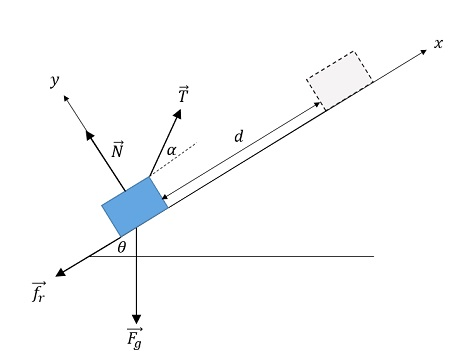# 10 kg crate is pulled 4.9 m up a 34 degree incline by a rope angled 16 degree above the incline....

## Question:

{eq}10\ kg {/eq} crate is pulled {eq}4.9\ m {/eq} up a {eq}34 {/eq} degree incline by a rope angled {eq}16 ^\circ {/eq} above the incline. The tension in the rope is {eq}125\ N {/eq}, and the crate's coefficient of kinetic friction on the incline is {eq}0.26 {/eq}.

How much work is done by:

a. Tension?

b. Gravity?

c. Normal Force?

d. What is the increase in thermal energy of the crate and incline?

## Mechanical work

The work performed by a force is defined as,

{eq}W_F=\displaystyle_{traj} \vec{F}\cdot d\vec{l} {/eq}.

If the force's value and direction (relative to the path) remains constant along the trajectory, the integral takes the simplified form,

{eq}W_F=\vec{F}\cdot \vec{d}=Fd\cos\alpha_{Fd} {/eq},

simply the scalar product of the force and the displacement. Notice this scalar product depends on the angle between the force and the displacement. If the angle is zero the work is maximum and for {eq}\alpha_{Fd}=90^\circ {/eq} it goes zero.

Let us start by representing all the interactions in a free-body diagram.The forces acting on the crate are:

{eq}\bullet {/eq} Tension, {eq}\vec{T} {/eq} directed forming an angle {eq}\alpha {/eq} relative to the positive {eq}x {/eq} axis.

{eq}\bullet {/eq} Friction, {eq}\vec{f}_r {/eq} directed along the negative {eq}x {/eq} axis.

{eq}\bullet {/eq} Normal force, {eq}\vec{N} {/eq} directed along the positive {eq}y {/eq} axis.

{eq}\bullet {/eq} Gravity, {eq}\vec{F}_g {/eq} with components along both axes. Forms an angle {eq}\theta {/eq} with the negative {eq}y {/eq} axis.

Since all the forces are constant the work can be determined from the expression,

{eq}W_{F}=\vec{F}\cdot \vec{d} {/eq},

where {eq}\vec{F} {/eq} stands for the force in question and {eq}\vec{d} {/eq} is the displacement of the crate.

a) The work of the tension force is,

{eq}\begin{align} W_{T}&=\vec{T}\cdot \vec{d}\\ &=Td\cos \alpha\\ &=125\;\rm \cdot 4.9\;\rm m \cos 16^\circ\\ &=\boxed{588.8 \;\rm J} \end{align} {/eq},

notice that the angle {eq}\alpha {/eq} formed by the rope and the displacement appears in the scalar product.

b) The work performed by the gravity force can be computed similarly. Yet, it is easier to use the definition of gravitational potential energy,

{eq}W_{F_g}=-\Delta E_{gp} {/eq},

the work of the gravity force equals minus the variation of the gravitational potential energy. Establishing the reference level at the initial height of the crate, the work of the gravity is,

{eq}W_{F_g}=-mg(h_f-h_0) {/eq},

with {eq}h_f=d\sin \theta {/eq} and {eq}h_0=0 {/eq}.

Subsituting,

{eq}\begin{align} W_{F_g}&=-mgd\sin\theta\\ &=-10\;\rm kg \cdot 9.8\;\rm m/s^2 \cdot 4.9 \;\rm m \cdot \sin 34^\circ\\ & =\boxed{-268.5 \;\rm J} \end{align} {/eq}.

c) The work performed by the normal force is zero. By definition {eq}\vec{N} {/eq} is perpendicular to the displacement, hence,

{eq}W_{N}=\vec{N}\cdot \vec{d}=Nd\cos 90^\circ=0 {/eq}.

d) Finally, the increase in thermal energy of the crate and incline equals the work performed by the frictional forces. In this case,

{eq}\begin{align} W_{f_r}&=\vec{f}_r\cdot \vec{d}\\ &=f_r d\cos180^\circ \end{align} {/eq}.

To calculate this work we need to determine the value of the friction force. From Newton's equation along the {eq}y {/eq} axis we obtain,

{eq}T_y+N-F_{gy}=0 {/eq},

substituting the projections in terms of the trigonometric functions,

{eq}N=mg\cos\theta-T\sin\alpha {/eq}.

The friction force is then,

{eq}f_r=\mu N=\mu (mg\cos\theta-T\sin\alpha) =0.26 \cdot (98\;\rm N\cdot 34^\circ -125 \;\rm N \cdot sin 16^\circ)=12.2 \;\rm N {/eq}.

Substituting for the work of the kinetic friction,

{eq}\begin{align} W_{f_r}&=12.2 \;\rm N \cdot 4.9\;\rm m \cdot \cos180^\circ\\ &=\boxed{-59.8 \;\rm J} \end{align} {/eq}.

The work performed by the friction, i.e., the increase in the internal energy of the system is {eq}-59.8 \;\rm J {/eq}.